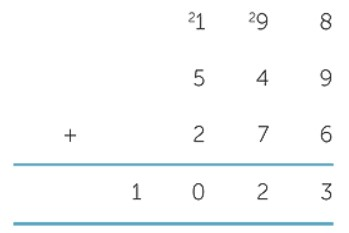Enter numbers in the input box and separate them using comma. Click the Calculate button to add these numbers using long addition calculator.

Give Us Feedback

Our long adding calculator is an online tool that adds whole numbers and decimal numbers and shows all the steps. It sums up all types of numbers as well as fractions, expressions, and exponents.## How to do long addition?

If you want to add long numbers without using a long sum calculator, follow the example given below.

Example: What is the sum of the following numbers?

1100, 894, 8923, 324.98

Step 1: Place all numbers under one another.

 1 1 0 0 8 9 2 3 8 9 4 + 3 2 4 . 9 8

Step 2: Start adding from right to left. Carry the right number to the next column if sum of a column is in double digit.

For example, if a sum of few numbers is 12, place 2 under the column and carry 1 to the next column.

 Carry 1 1 1 1 0 0 8 9 4 8 9 2 3 + 3 2 4 . 9 8 1 1 2 4 1 . 9 8

So, 1100 + 894 + 8923 + 324.98 = 11241.98

Use our summation calculator if you need to take sum of a series for an expression over a predetermined range.

### Math Tools